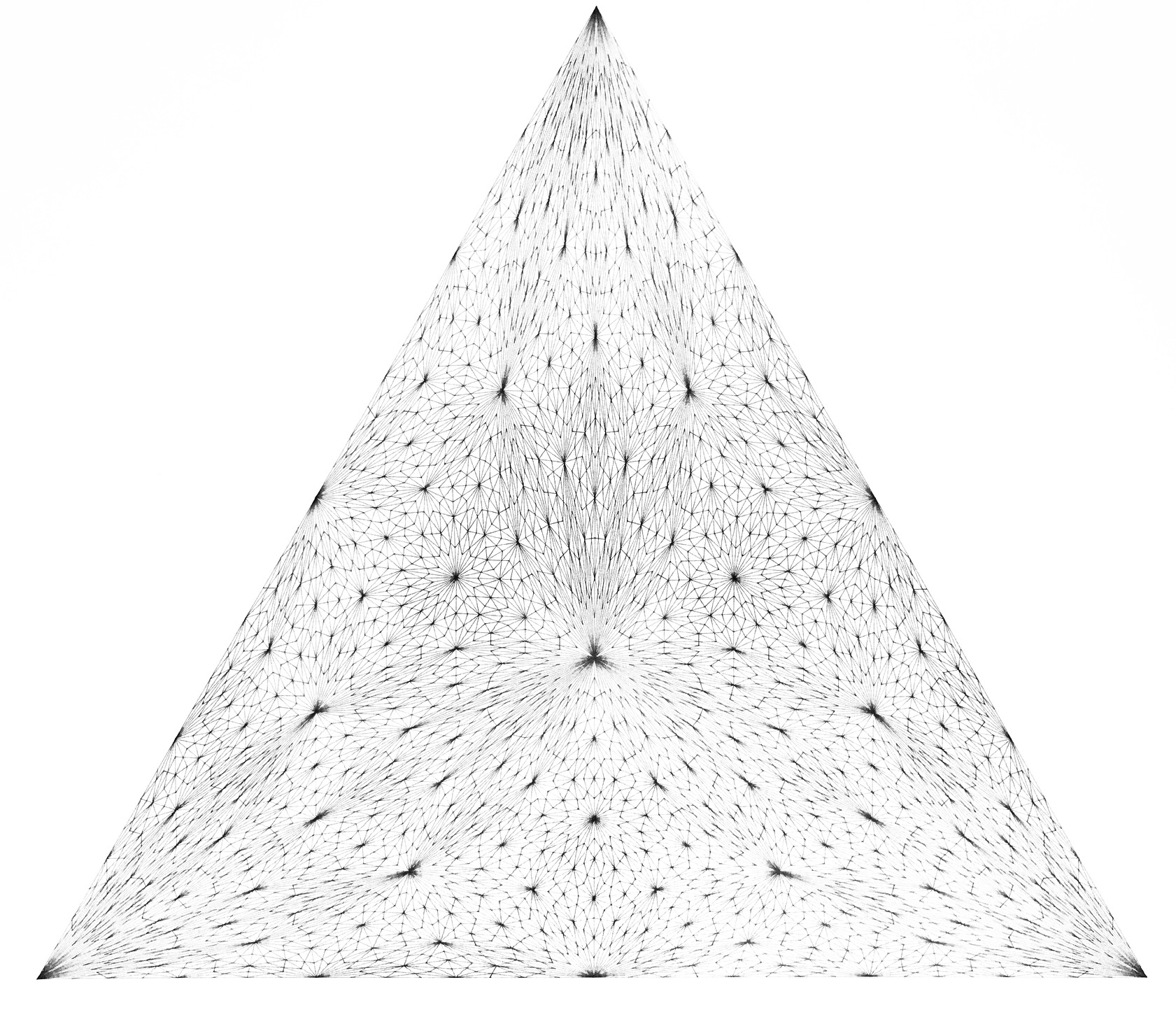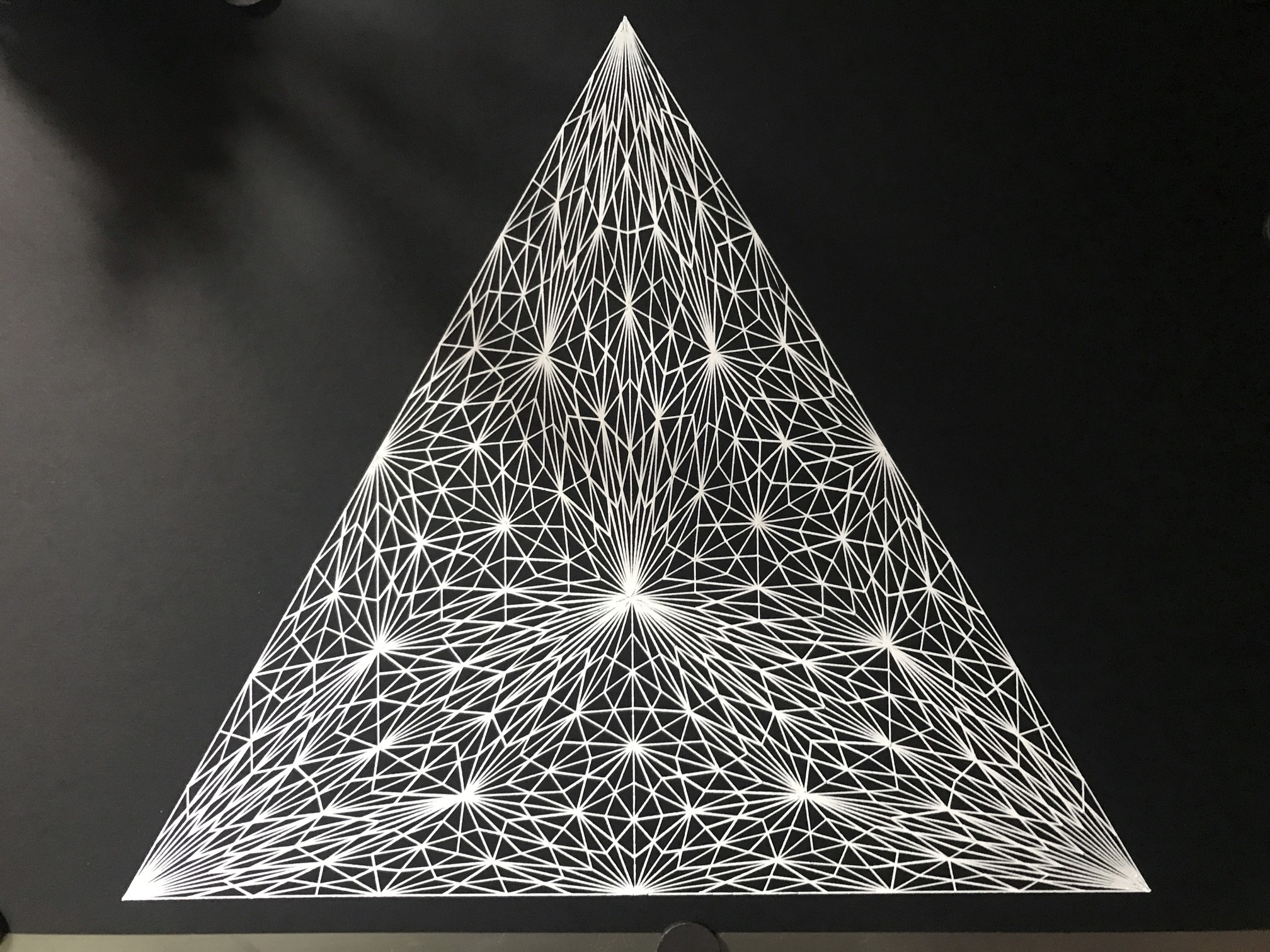When you draw a triangle and then draw the medians (the line that connects an angle with the center of the opposite side) you get six triangles. You can repeat this process with all emerging triangles recursively. And then plot this.

####Triangles in triangles, 7 recursive steps, black on white. Equilateral triangle

• 7 recursive steps. About 50,000 small triangles.
• Plotted on CANSON® XL® Bristol Bristolpapier A3.
• Black pigmented pen
• Dimensions: 27.5×27.5 cm

####Triangles in triangles, 6 recursive steps, white on black. Equilateral triangle

• 6 recursive steps. About 10,000 small triangles.
• Plotted on Fabriano BLACK BLACK paper, 300g  A4.
• White pen (Mitsubishi Uni-ball)
• Dimensions: 27.5×27.5 cm